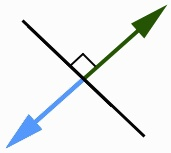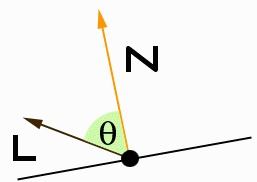IntroductionI'll try to make this one a quickie. A fairly common question that I see on newsgroups or hear from newbie 3D coders is "what are vertex normals?" and the questions that follow (such as how to calculate them, what you can do with them, why you would do this, etc). I'll try to explain a bit about them really quickly and show a couple uses that may be of interest to you. They're not very difficult to understand at all, and if you understand "normal" normals (eh eh), this should be a breeze.

 Vertex NormalsHopefully you know what normals are. Here's a quick diagram to illustrate a normal in 2D. As you can see, they're directions perpendicular to the original 'surface' (our line), and extend on both sides (forming 90 degree angles).The same applies in 3-space such that the normals are orthogonal to the original 'line'. Imagine for example the line as an infinite plane in 3D. The normal is a vector that is orthogonal to that plane. They're used for a lot of things in 3D calculations such as backface culling (by checking what direction a polygon is facing, in other words which way the polygon's normal is pointing). So that's nice, but...

Say you want to work with lighting and shading some of your objects. Check out the following (attempt at a) picture:In many lighting computations (there are many local reflection models to choose from, so I won't mention any in particular), one would use the angle between the normal and the light vector to determine how much light is being reflected (as well as mirror angles, view angles, etc -- this depends on what kind of lighting you're doing), thus labelling the surface with a certain 'brightness'. This document isn't about lighting, so I won't get into much about the properties of light or how things reflect, but you may have gathered that using face normals (the normal of a polygon 'face'), we can only get a particular 'brightness' for an entire polygon. That would be nice if we only wanted to do flat shading, but that's painfully boring for most games and demos. Our goal is to find a normal for EACH vertex that we can use. This would let us, for example, interpolate the 'brightness' across the surface for use with techniques like gouraud shading, or even interpolate the normal itself for use with phong shading.

So how do we get these magical normals? Because normals don't exactly exist at the vertices, I believe some people call them vertex psuedo-normals. We can get a pretty good idea of what they should be by simply averaging the surrounding face normals. That is to say -- the normals of the polygons that share the particular vertex whose psuedo-normal we are trying to compute. Not too bad, eh? This works ok for typical objects like spheres, torii, and so on -- as long as the polygon arrangements offer a decent representation of the actual object they're representing, this method should do fine. So now like the light angle picture above, you can use the vertex normal instead of the face normal to calculate the brightness at any particular vertex. Hopefully you see why that is a good thing. Below is some general psuedo-code.

Psuedo-Code:

 ``` // Calculating vertex normals using a vertex list and polygon faces (vertex indices); loop through your vertex list { clear this_vertex_normal; // initialized to [0,0,0] loop through your face lists { for each face vertex, check if its the same as the vertex as in our current outer vertex loop. If it is, { this_vertex_normal += this_face.normal; } } this_vertex_normal.Normalize(); } ```

 ClosingThat's pretty much it. I haven't seen many uses for vertex normals besides things like lighting or fake phong shading (ie using the vertex normals as texture coordinates with a 'phong map' as a texture), but if you get really creative -- I'm sure you can find some other uses for them. Keep in mind that they are in fact just approximations and aren't always guaranteed to be what you're looking for. As with all normals, double check that they're pointing the right way -- normals can be tricky like that in 3D. One more caveat: don't forget to rotate them just like you do your ordinary vertices. I hope that cleared something up. If you spotted any errors, let me know.